# Elasticity Lecture 5 Price Elasticity of Demand Slope

• Slides: 28
Download presentation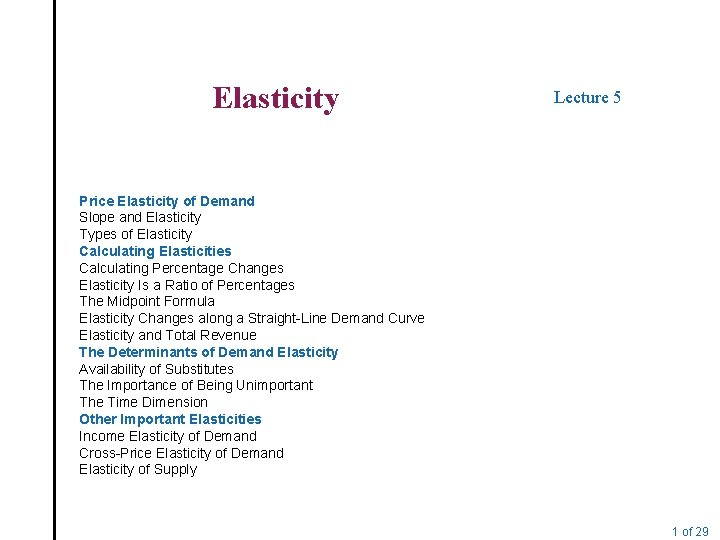Elasticity Lecture 5 Price Elasticity of Demand Slope and Elasticity Types of Elasticity Calculating Elasticities Calculating Percentage Changes Elasticity Is a Ratio of Percentages The Midpoint Formula Elasticity Changes along a Straight-Line Demand Curve Elasticity and Total Revenue The Determinants of Demand Elasticity Availability of Substitutes The Importance of Being Unimportant The Time Dimension Other Important Elasticities Income Elasticity of Demand Cross-Price Elasticity of Demand Elasticity of Supply 1 of 29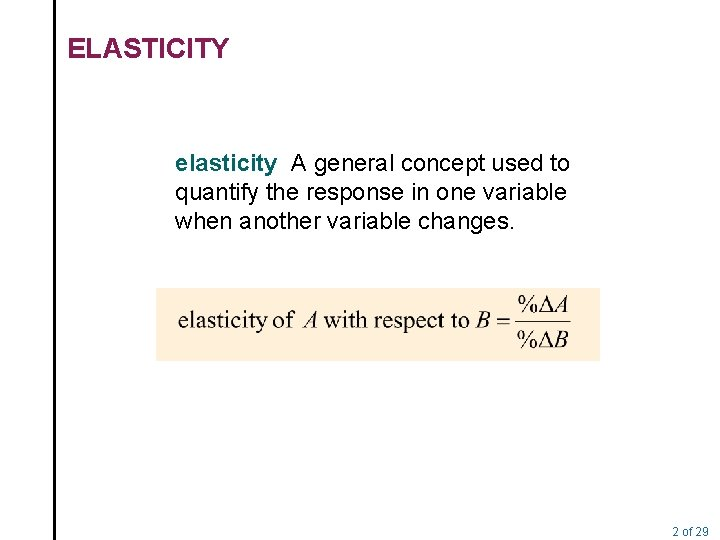ELASTICITY elasticity A general concept used to quantify the response in one variable when another variable changes. 2 of 29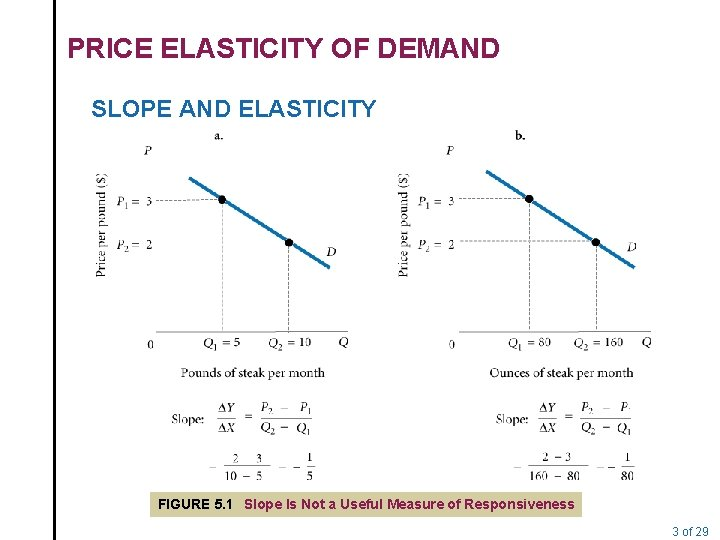PRICE ELASTICITY OF DEMAND SLOPE AND ELASTICITY FIGURE 5. 1 Slope Is Not a Useful Measure of Responsiveness 3 of 29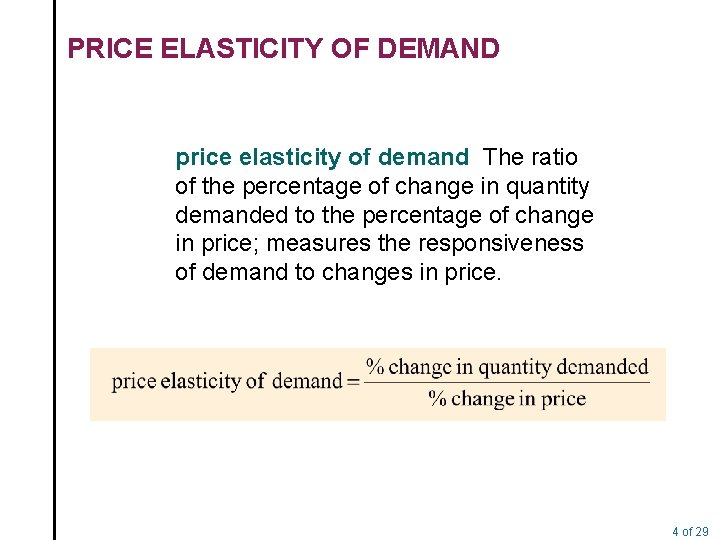PRICE ELASTICITY OF DEMAND price elasticity of demand The ratio of the percentage of change in quantity demanded to the percentage of change in price; measures the responsiveness of demand to changes in price. 4 of 29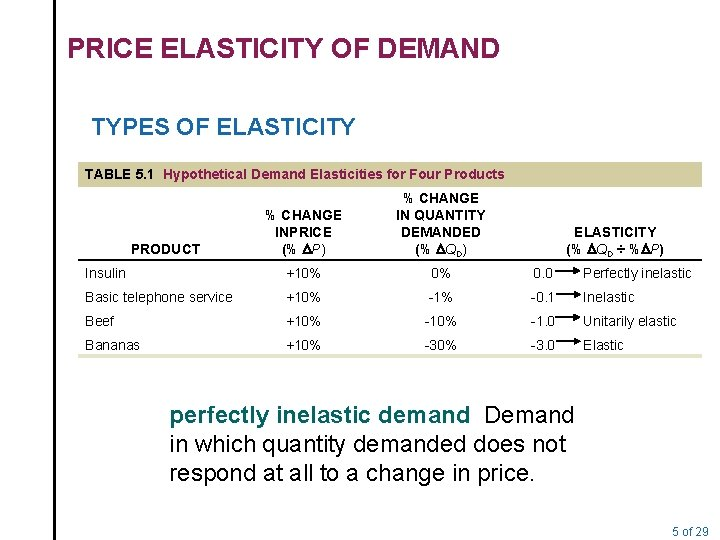PRICE ELASTICITY OF DEMAND TYPES OF ELASTICITY TABLE 5. 1 Hypothetical Demand Elasticities for Four Products % CHANGE INPRICE (% DP) % CHANGE IN QUANTITY DEMANDED (% DQD) Insulin +10% 0% 0. 0 Perfectly inelastic Basic telephone service +10% -1% -0. 1 Inelastic Beef +10% -1. 0 Unitarily elastic Bananas +10% -3. 0 Elastic PRODUCT ELASTICITY (% DQD ÷ %DP) perfectly inelastic demand Demand in which quantity demanded does not respond at all to a change in price. 5 of 29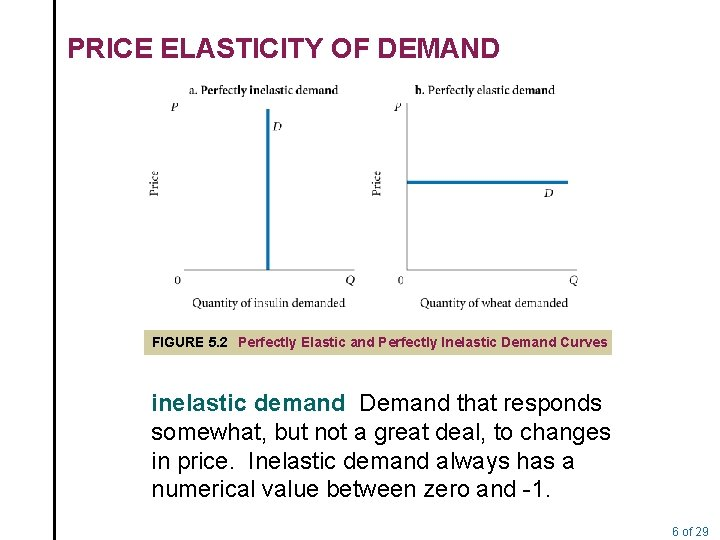PRICE ELASTICITY OF DEMAND FIGURE 5. 2 Perfectly Elastic and Perfectly Inelastic Demand Curves inelastic demand Demand that responds somewhat, but not a great deal, to changes in price. Inelastic demand always has a numerical value between zero and -1. 6 of 29PRICE ELASTICITY OF DEMAND A warning: You must be very careful about signs. Because it is generally understood that demand elasticities are negative (demand curves have a negative slope), they are often reported and discussed without the negative sign. For example, a technical paper might report that the demand for housing “appears to be inelastic with respect to price, or less than 1 (0. 6). ” What the writer means is that the estimated elasticity is -. 6, which is between zero and -1. Its absolute value is less than 1. unitary elasticity A demand relationship in which the percentage change in quantity of a product demanded is the same as the percentage change in price in absolute value (a demand elasticity of -1). 7 of 29PRICE ELASTICITY OF DEMAND elastic demand A demand relationship in which the percentage change in quantity demanded is larger in absolute value than the percentage change in price (a demand elasticity with an absolute value greater than 1). perfectly elastic demand Demand in which quantity drops to zero at the slightest increase in price. 8 of 29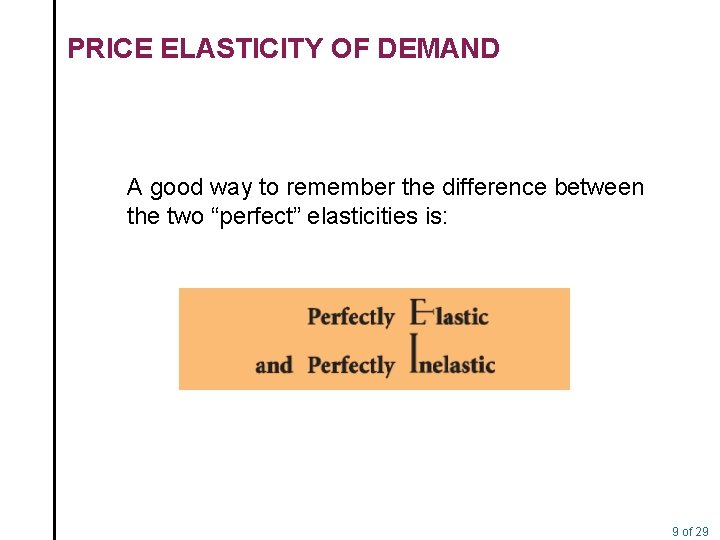PRICE ELASTICITY OF DEMAND A good way to remember the difference between the two “perfect” elasticities is: 9 of 29CALCULATING ELASTICITIES CALCULATING PERCENTAGE CHANGES To calculate percentage change in quantity demanded using the initial value as the base, the following formula is used: 10 of 29CALCULATING ELASTICITIES We can calculate the percentage change in price in a similar way. Once again, let us use the initial value of P— that is, P 1—as the base for calculating the percentage. By using P 1 as the base, the formula for calculating the percentage of change in P is simply: 11 of 29CALCULATING ELASTICITIES ELASTICITY IS A RATIO OF PERCENTAGES Once all the changes in quantity demanded and price have been converted into percentages, calculating elasticity is a matter of simple division. Recall the formal definition of elasticity: 12 of 29CALCULATING ELASTICITIES THE MIDPOINT FORMULA midpoint formula A more precise way of calculating percentages using the value halfway between P 1 and P 2 for the base in calculating the percentage change in price, and the value halfway between Q 1 and Q 2 as the base for calculating the percentage change in quantity demanded. 13 of 29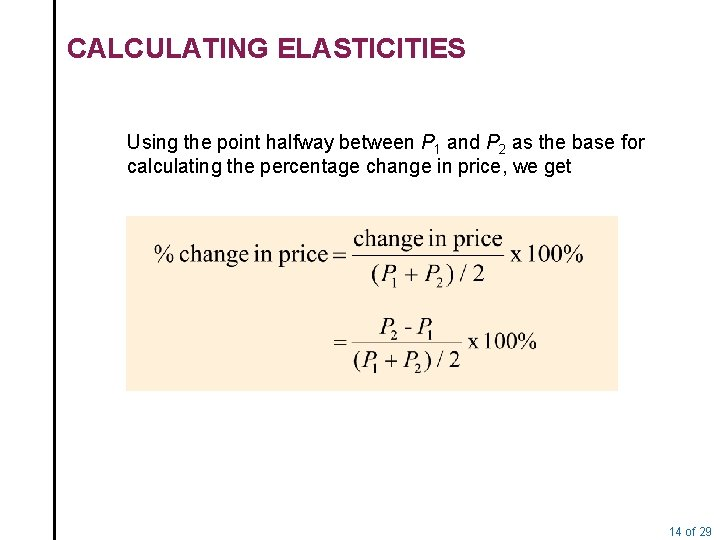CALCULATING ELASTICITIES Using the point halfway between P 1 and P 2 as the base for calculating the percentage change in price, we get 14 of 29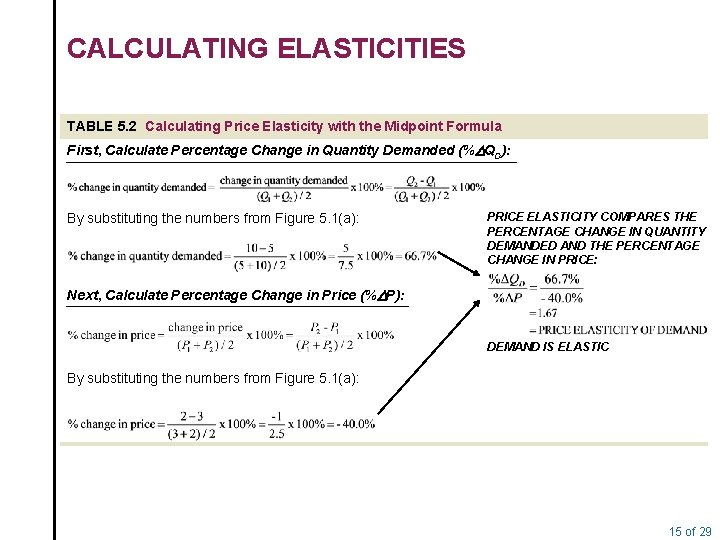CALCULATING ELASTICITIES TABLE 5. 2 Calculating Price Elasticity with the Midpoint Formula First, Calculate Percentage Change in Quantity Demanded (%DQD): By substituting the numbers from Figure 5. 1(a): PRICE ELASTICITY COMPARES THE PERCENTAGE CHANGE IN QUANTITY DEMANDED AND THE PERCENTAGE CHANGE IN PRICE: Next, Calculate Percentage Change in Price (%DP): DEMAND IS ELASTIC By substituting the numbers from Figure 5. 1(a): 15 of 29CALCULATING ELASTICITIES ELASTICITY CHANGES ALONG A STRAIGHTLINE DEMAND CURVE TABLE 5. 3 Demand Schedule for Office Dining Room Lunches PRICE QUANTITY DEMANDED (PER LUNCH) (LUNCHES PER MONTH) \$11 10 9 8 7 6 5 4 3 2 1 0 0 2 4 6 8 10 12 14 16 18 20 22 FIGURE 5. 3 Demand Curve for Lunch at the Office Dining Room 16 of 29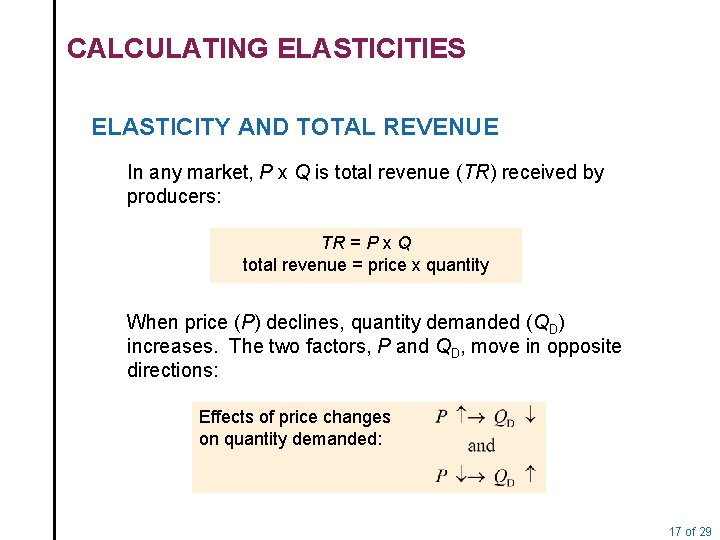CALCULATING ELASTICITIES ELASTICITY AND TOTAL REVENUE In any market, P x Q is total revenue (TR) received by producers: TR = P x Q total revenue = price x quantity When price (P) declines, quantity demanded (QD) increases. The two factors, P and QD, move in opposite directions: Effects of price changes on quantity demanded: 17 of 29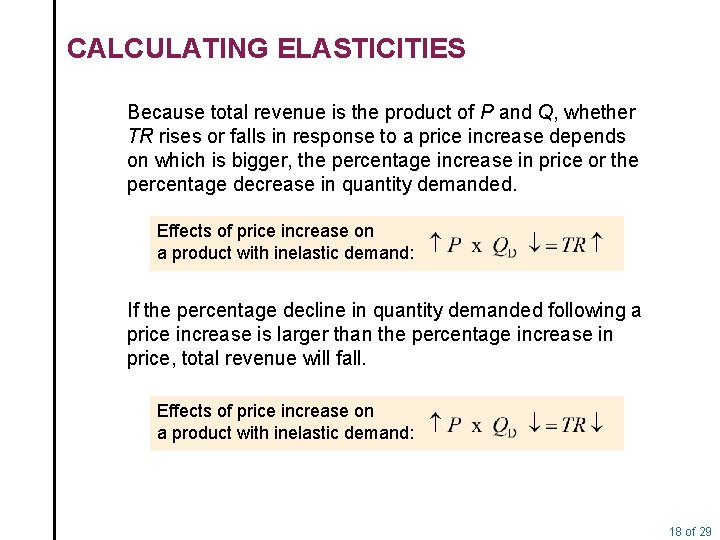CALCULATING ELASTICITIES Because total revenue is the product of P and Q, whether TR rises or falls in response to a price increase depends on which is bigger, the percentage increase in price or the percentage decrease in quantity demanded. Effects of price increase on a product with inelastic demand: If the percentage decline in quantity demanded following a price increase is larger than the percentage increase in price, total revenue will fall. Effects of price increase on a product with inelastic demand: 18 of 29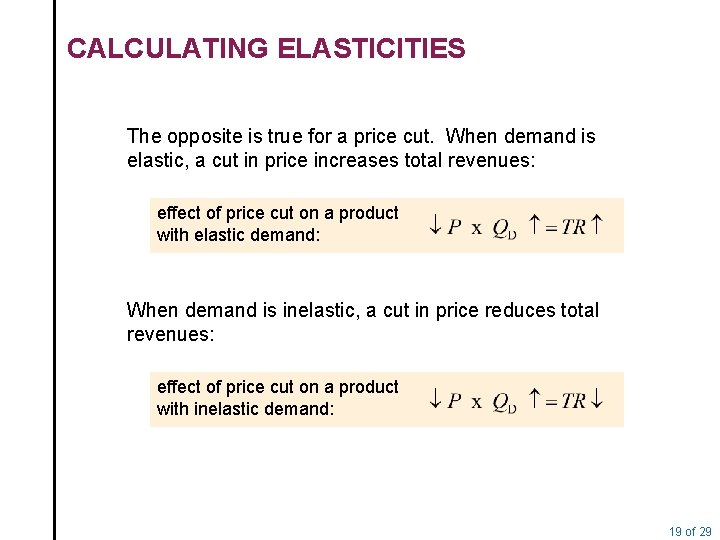CALCULATING ELASTICITIES The opposite is true for a price cut. When demand is elastic, a cut in price increases total revenues: effect of price cut on a product with elastic demand: When demand is inelastic, a cut in price reduces total revenues: effect of price cut on a product with inelastic demand: 19 of 29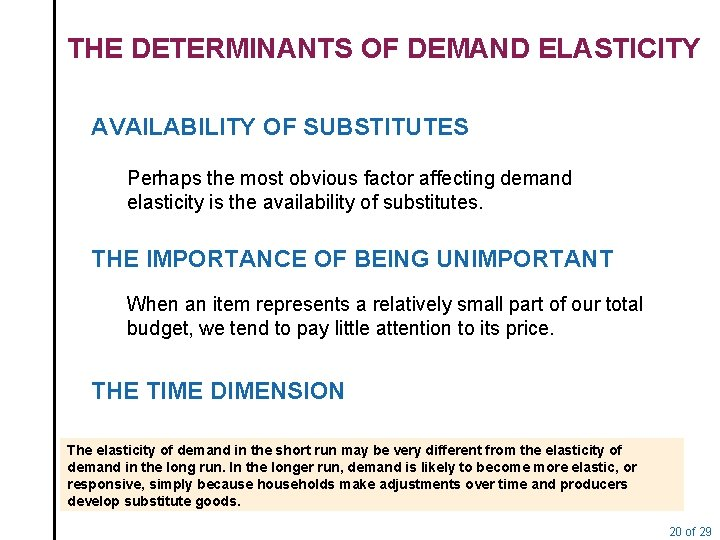THE DETERMINANTS OF DEMAND ELASTICITY AVAILABILITY OF SUBSTITUTES Perhaps the most obvious factor affecting demand elasticity is the availability of substitutes. THE IMPORTANCE OF BEING UNIMPORTANT When an item represents a relatively small part of our total budget, we tend to pay little attention to its price. THE TIME DIMENSION The elasticity of demand in the short run may be very different from the elasticity of demand in the long run. In the longer run, demand is likely to become more elastic, or responsive, simply because households make adjustments over time and producers develop substitute goods. 20 of 29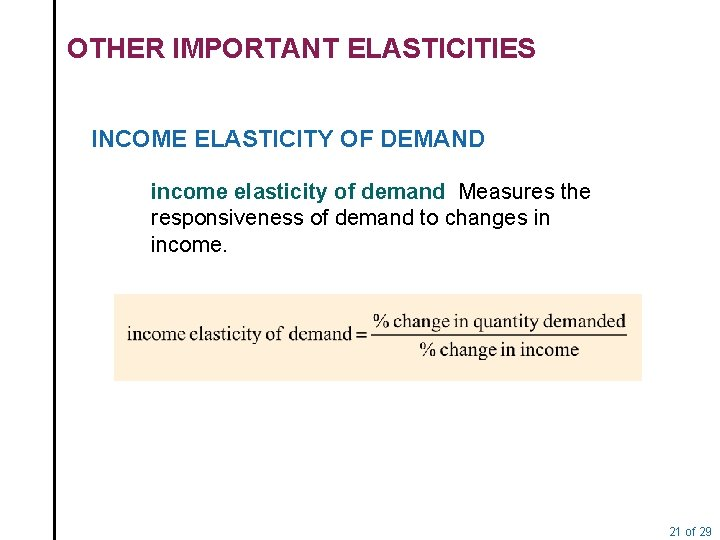OTHER IMPORTANT ELASTICITIES INCOME ELASTICITY OF DEMAND income elasticity of demand Measures the responsiveness of demand to changes in income. 21 of 29OTHER IMPORTANT ELASTICITIES CROSS-PRICE ELASTICITY OF DEMAND cross-price elasticity of demand A measure of the response of the quantity of one good demanded to a change in the price of another good. 22 of 29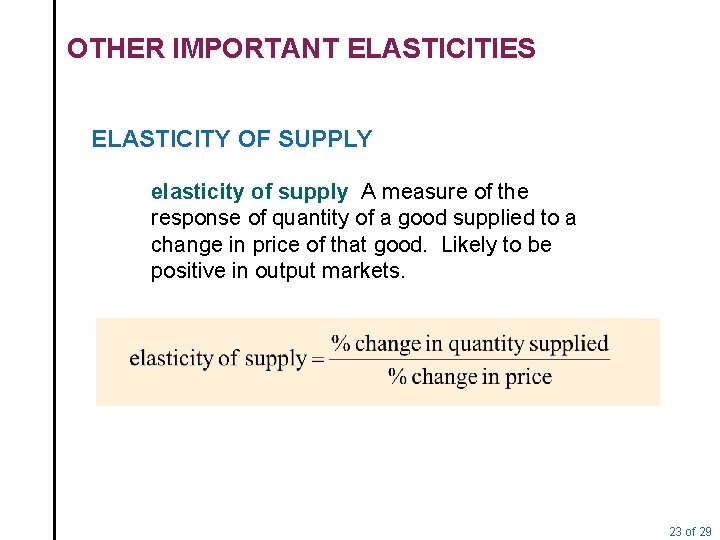OTHER IMPORTANT ELASTICITIES ELASTICITY OF SUPPLY elasticity of supply A measure of the response of quantity of a good supplied to a change in price of that good. Likely to be positive in output markets. 23 of 29OTHER IMPORTANT ELASTICITIES elasticity of labor supply A measure of the response of labor supplied to a change in the price of labor. 24 of 29REVIEW TERMS AND CONCEPTS cross-price elasticity of demand elasticity of labor supply elasticity of supply income elasticity of demand inelastic demand midpoint formula perfectly elastic demand perfectly inelastic demand price elasticity of demand unitary elasticity 25 of 29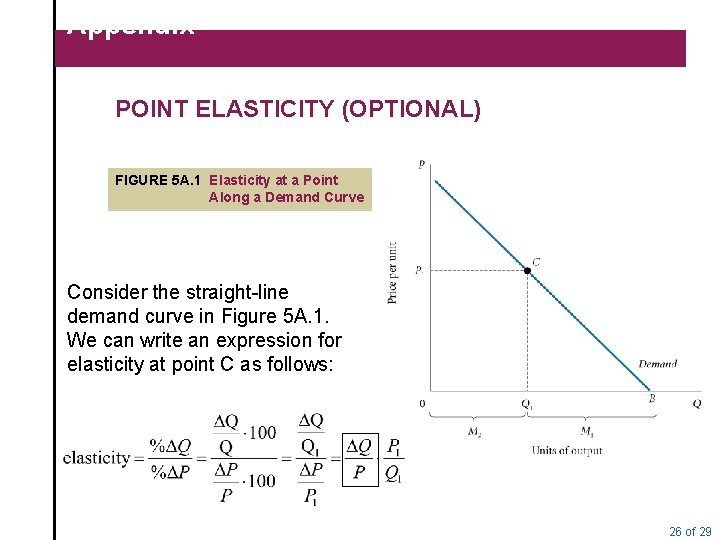Appendix POINT ELASTICITY (OPTIONAL) FIGURE 5 A. 1 Elasticity at a Point Along a Demand Curve Consider the straight-line demand curve in Figure 5 A. 1. We can write an expression for elasticity at point C as follows: 26 of 29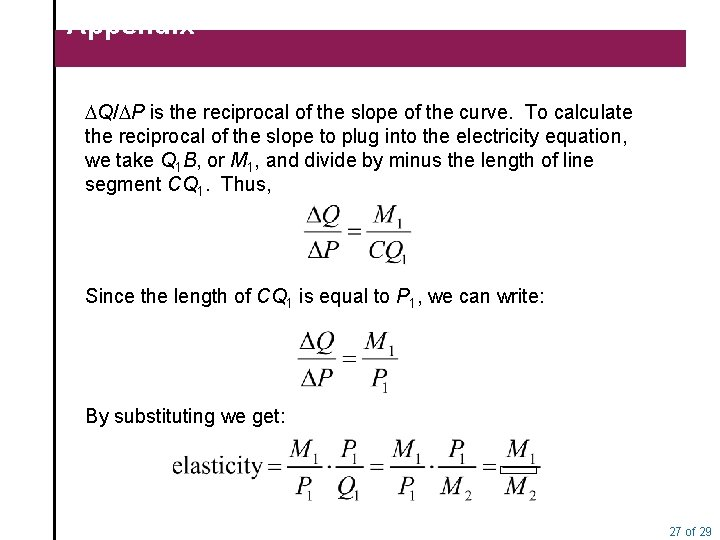Appendix DQ/DP is the reciprocal of the slope of the curve. To calculate the reciprocal of the slope to plug into the electricity equation, we take Q 1 B, or M 1, and divide by minus the length of line segment CQ 1. Thus, Since the length of CQ 1 is equal to P 1, we can write: By substituting we get: 27 of 29Appendix FIGURE 5 A. 2 Point Elasticity Changes Along a Demand Curve 28 of 29